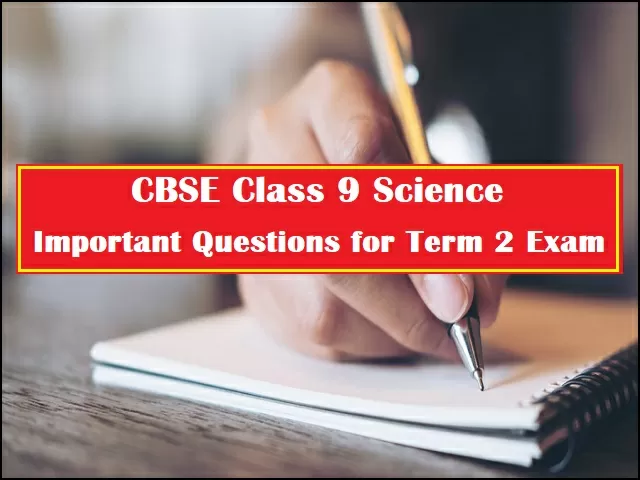# CBSE Class 9 Science Important Long & Short Answer Questions for Term 2 Exam 2022: Best for Quick Revision

## Important questions for CBSE Class 9 Science are provided here to help students prepare chapter-wise questions of 2, 3 and 4 marks for the upcoming Term 2 Exam. All questions are prepared by the exam experts.CBSE Class 9 Science Important Questions for Term 2 Exam 2022

CBSE Class 9 Science 2022: Jagran Josh presents here important practice questions for Class 9 Science that must be prepared for the upcoming CBSE Term 2 Exam 2022. The chapter-wise questions of 2, 3 and 4 marks are prepared by the exam experts to cover the important topics occurring in CBSE Class 9 Science Term 2 Syllabus. Students who want to score above 90% marks in their CBSE Class 9 Science Term 2 Exam must solve the important questions given below.

## CBSE Class 9 Science Important Very Short Answer Type Questions (2 Marks)

Some of the questions are mentioned below:

Q. Write the full form of WHO.

Q. Name two non-infectious diseases.

Q. Write two water-borne diseases.

Q. Write the difference between acute and chronic disease.

Q. Write the expanded form of AIDS.

Q. What is the difference between ‘Being healthy’ and ‘Disease free’?

Q. Name two diseases that can be prevented by using vaccine.

Q. Name the methods for treatment of infectious diseases.

Q. Name the organism that causes kala azar.

## CBSE Class 9 Science Important Short Answer Type Questions (3 Marks)

Some of the questions are mentioned below:

Q. What is conservation of energy? Explain with an example.

Q. What do you understand by kinetic energy? Write its formula.

Q. On what factors does the kinetic energy of a body depends?

Q. What happens to potential energy of a body when its height is doubled?

Q. How many joules are there in 1 Kilowatt hour?

Q. Derive the formula for potential energy.

Q. If an electric iron of 200 W is used for 30 minutes everyday, find electric energy consumed in the month of April.

## CBSE Class 9 Science Important Case Based Questions (4 Marks)

Some of the questions are mentioned below:

The concept of work in physics is much more narrowly defined than the common use of the word. Work is done on an object when an applied force moves it through a distance. In our everyday language, work is related to expenditure of muscular effort, but this is not the case in the language of physics. A person that holds a heavy object does no physical work because the force is not moving the object through a distance. Work, according to the physics definition, is being accomplished while the heavy object is being lifted but not while the object is stationary. Another example of the absence of work is a mass on the end of a string rotating in a horizontal circle on a frictionless surface. The centripetal force is directed toward the center of the circle and, therefore, is not moving the object through a distance; that is, the force is not in the direction of motion of the object. (However, work was done to set the mass in motion.) Mathematically, work is W = F×x, where F is the applied force and x is the distance moved, that is, displacement. Work is a scalar. The SI unit for work is the joule (J), which is newton‐meter or kg m/s2.

1. When a body falls freely towards the earth, then its total energy:

(a) increases

(b) decreases

(c) remains constant

(d) first increases and then decreases

2. A car is accelerated on a levelled road and attains a velocity 4 times of its initial velocity. In this process the potential energy of the car:

(a) does not change

(b) becomes twice to that of initial

(c) becomes 4 times that of initial

(d) becomes 16 times that of initial

3. In case of negative work the angle between the force and displacement is:

(a) 0

(b) 450

(c) 900

(d) 1800

4. An iron sphere of mass 10 kg has the same diameter as an aluminium sphere of mass is 3.5 kg. Both spheres are dropped simultaneously from a tower. When they are 10m above the ground, they have the same:

(a) acceleration

(b) momenta

(c) potential energy

(d) kinetic energy

1.(c) remains constant

2.(a) does not change

3.(d) 1800

4.(a) acceleration

Also, check

CBSE Class 9 Science Best Study Material for Term 2 Exam 2022

Get here latest School, CBSE and Government Jobs notification in English and Hindi for Sarkari Naukari and Sarkari Result. Download the Jagran Josh Sarkari Naukri App.

## Trending Categories

This website uses cookie or similar technologies, to enhance your browsing experience and provide personalised recommendations. By continuing to use our website, you agree to our Privacy Policy and Cookie Policy.